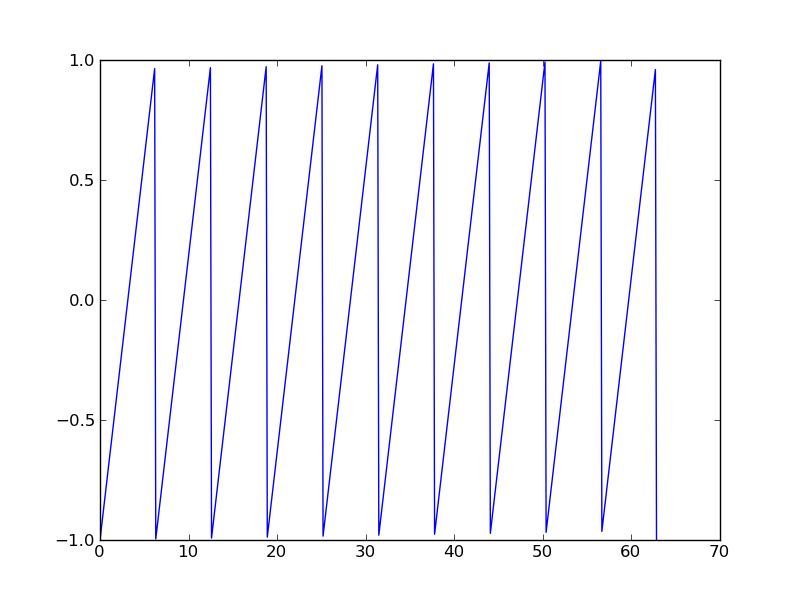# scipy.signal.sawtooth¶

scipy.signal.sawtooth(t, width=1)

Return a periodic sawtooth waveform.

The sawtooth waveform has a period 2*pi, rises from -1 to 1 on the interval 0 to width*2*pi and drops from 1 to -1 on the interval width*2*pi to 2*pi. width must be in the interval [0,1].

Parameters : t : array_like Time. width : float, optional Width of the waveform. Default is 1. y : ndarray Output array containing the sawtooth waveform.

Examples

```>>> import matplotlib.pyplot as plt
>>> x = np.linspace(0, 20*np.pi, 500)
>>> plt.plot(x, sp.signal.sawtooth(x))
```#### Previous topic

scipy.signal.gausspulse

#### Next topic

scipy.signal.square